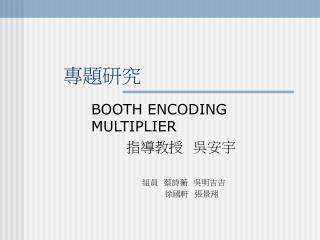DownloadDownload Presentation# 專題研究

Download Presentation## 專題研究

- - - - - - - - - - - - - - - - - - - - - - - - - - - E N D - - - - - - - - - - - - - - - - - - - - - - - - - - -
##### Presentation Transcript

1. 專題研究 BOOTH ENCODING MULTIPLIER 指導教授 吳安宇 組員 蔡詩蘅 吳明吉吉 徐國軒 張景翔

2. 1.Booth encoding • What is Booth Encoding ? • Modified Booth Encoding • Sign Extension-compensation vector • Original Multiplier Structure

3. 2.Power Dissipation • Transition probability • MSD Property • Proposed Multiplier Structure

4. What is Booth Encoding ? • Y = -2n-1yn-1 + 2n-2yn-2+…+20y0 =-2n-1(yn-2 -yn-1 ) + 2n-2(yn-3 -yn-2) +……+20(y1 –y0)

5. Modified Booth Encoding • Y = -2n-1yn-1 + 2n-2yn-2+…+20y0 = 2n-2(2yn-1 - 2yn – yn-1 + yn-2) +…… + 20(-2y1+y0) = 2n-2(- 2yn + yn-1 + yn-2 ) +……

6. Radix-4 Modified Booth Encoding Table Y2i+1 Y2i Y2i-1 | Si ---------------------------------------- 0 0 0 | +0 0 0 1 | +1 0 1 0 | +1 0 1 1 | +2 1 0 0 | - 2 1 0 1 | - 1 1 1 0 | - 1 1 1 1 | +0

7. Booth Multiplier Structure

8. Sign-generate Sign extension

9. Correction Factor

10. Example

11. Reduced Transitions

12. Encoding Operation

13. Architecture of Booth-encoding Multiplier

14. The Power Dissipation • Power = 0.5CLVDD2fCLKESW ESW :The switching activity =>The probability of a transition within a clock cycle

15. Transition probability • PΔ(s) = 2p(s)(1-p(s)) p(s)=probability of s being non- zero Transition:May cause glitches in combinatorial circuits=> High switch probability->Short logic depth

16. The One Probability of A Carry Signal • P(C1) = p(Qm)p(Qn) Qm,Qn are the two partial-products added in the first adder row

17. Carry output of k-th adder row • P(Ck) = 0.5p(Ck-1) + 0.5p(Qi) Ck :a carry out of the k-th adder row Qi :a partial product added into the k-th adder row (P(CARRY)=p1p2+p2p3+p3p1-2p1p2p3 when p1=0.5)

18. MSD • For a signal that has a smaller magnitude than its wordlength • 000….xxxxx…. • 111….xxxxx…. • Repeat sign-extension bits • Booth encoding (Si) as +0

19. A Signal Property • P(Qi) = p(X)p(Si) • p(X)=0.5 for random multiplicand • The MSD has low p(Si) and hence low p(Qi) • P(Qi)<0.5, P(Qi) implies PΔ(Qi) • Fist add the MSD partial-product in a carry-save array for reduced switching

20. Proposed Architecture of Booth-encoding Multiplier